[Index]

This document is also available as plain text and pdf.

It was last exported on 2020-01-23 Thu 18:04.

# Generative Art with Context Free Grammars

## Theory

Context free grammars are a concept originating in linguistics but widely used in (theoretical) computer science.

For this section, I'm going to use a bunch of mathematical notation, but I'll try to explain everything as it comes along.

A set is collection of elements where each element appears only once. The set of the number from one to four can be written as $$\{1, 2, 3, 4\}$$.

An alphabet is a set with a finite number of elements (the natural numbers 1, 2, 3, …, would be an example for an infinite set).

A context free grammar has four components:

1. An alphabet $$T$$ of terminals (read: “things where the expansion terminates”)
2. An alphabet $$N$$ of non-terminals (read: “things that can expand into other things”)
3. A set $$P$$ of production rules
4. The start symbol $$S \in N$$, an element of the set of non-terminals

It's important that the terminal and non-terminal alphabets are disjoint, i.e. there is no element that is part of both sets.

Production rules have the form $$N \to (N \cup T)^\star$$. $$(N \cup T)$$ can be read as “Combine the sets $$T$$ and $$N$$”, $$^\star$$ is called Kleene star and can be read as “repeated zero or more times”.

The part on the right of the $$\to$$ is called right hand side, or RHS, the part on the left left hand side or LHS.

A grammar is expanded starting with the start symbol and then replacing each non-terminal with the RHS of one of its rules.

Time for a simple example:

• $$T = \{a\}$$
• $$N = \{A\}$$
• $$P = \{A \to BBa, B \to bb\}$$
• $$S = A$$

Starting from $$S$$, the grammar expands into $$BBa$$, then each $$B$$ expands into $$bb$$, so the end result is $$bbbba$$.

There can be multiple rules with the same right hand side, so there are (possibly infinitely) many sequences a grammar can expand to.

• $$T = \{a\}$$
• $$N = \{A\}$$
• $$P = \{A \to Aa, A \to \varepsilon\}$$
• $$S = A$$

$$\varepsilon$$ is the notation for an empty sequence, so when expanding $$A \to \varepsilon$$, the $$A$$ just disappears.

Expanding this grammar, we have two options, either expand $$A$$ to $$Aa$$ or to $$\varepsilon$$.

Expanding $$Aa$$ further, we have two options again, either expand it to $$Aaa$$ or to $$\varepsilon a = a$$.

This can be repeated infinitely many times, so the grammar produces sequences of $$a$$ of any length. (Remember the Kleene star? We can use it to write that as $$a^\star$$ instead).

Just expanding grammars into sequences of strings quickly gets boring. Terminals don't have to be characters, they can be (interpreted as) anything at all, for example functions that draw geometric shapes.

## Why CFGs?

The Chomsky hierarchy defines four levels of formal grammars:

1. Unrestricted (most powerful)
2. Context Sensitive
3. Context Free
4. Regular (simplest)

Usually, the problem is checking whether a word belongs to a grammar.

Here, we only care about generating random expansions of a grammar. For this CFGs are a good choice because random expansion is easy to implement and they are not as limited as regular grammars.

## Turtle Grammars

One easy way to visualize the output of the expansion of a grammar is to interpret it as instructions for a turtle-graphics program.

For the following examples, I'm using only three commands:

1. move forward
2. turn left / right
3. scale (reduce the size of each forward step)

The expansions of grammars can be infinitely long, so we need a mechanism to prevent this from happening. I'm simply limiting the depth of the expansion to some value, after that all non-terminals are ignored.

### Iteration

Let's start with a simple turtle rule system, to see how iteration can be implemented:

grammar.add_non_terminal('S', [
lambda turtle: turtle.forward(),
lambda turtle: turtle.turn_right(5),
lambda turtle: turtle.scale_by(0.99),
'S'
])

grammar.expand('S', turtle, 300)


For the examples, I'll be using a small python framework I've created instead of mathematical notation. If you want to follow along, download the code from https://github.com/l3kn/generative_cfg.

The main element of this framework is a Grammar. Non-terminals are just lists of either strings to reference another non-terminal or (anonymous) functions as terminals.

These terminal functions can act on some kind of state, in this case a turtle graphics object turtle.

Using grammar.add_non_terminal(name, body) new non-terminals can be added to the grammar.

A grammar can be expanded using the method grammar.expand(start_symbol, state, depth).

Now it should be clear how the code above produces the image below, the non-terminal $$S$$ makes the turtle move forward, turns it to the right by 5 degrees, and then reduces its scale by a factor of $$0.99$$.

Then the grammar is expanded to a depth of 300 using $$S$$ as start symbol.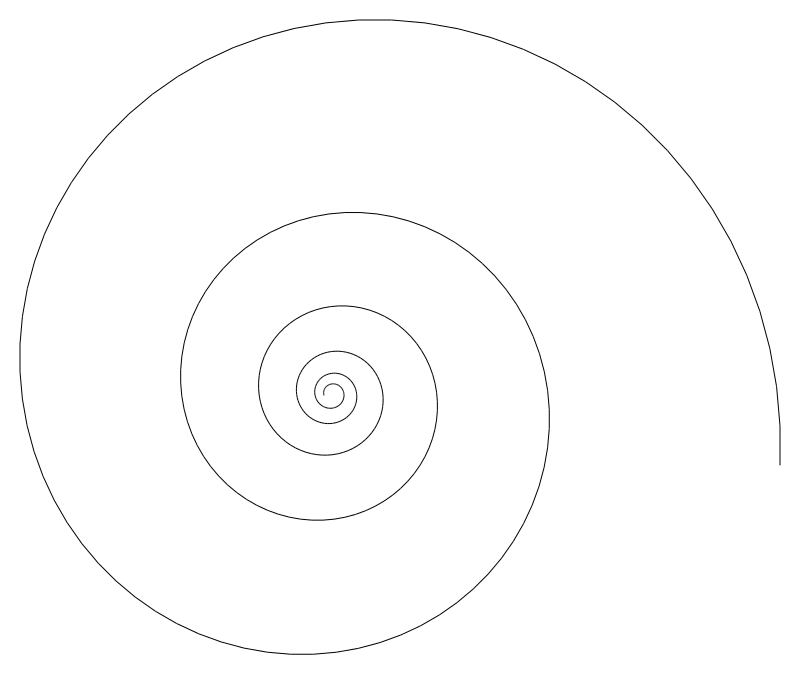Changing the rotation angle from 5 to 89.5 degrees leads to a very different result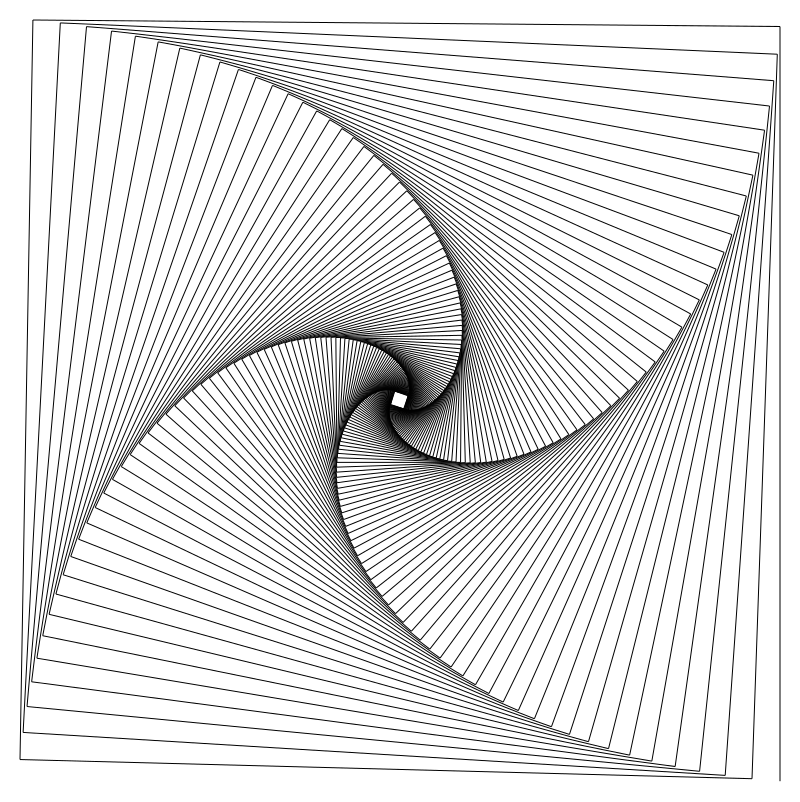### Branching 1

from generative_cfg import *

backend = SVGBackend()
turtle = Turtle(backend)
grammar = Grammar()
scale = 0.6

lambda t: t.store(),
lambda t: t.turn_right(45),
lambda t: t.forward(),
lambda t: t.scale(scale),
'Tree',
lambda t: t.restore(),
lambda t: t.turn_left(45),
lambda t: t.forward(),
lambda t: t.scale(scale),
'Tree',
])

grammar.expand('Tree', turtle, 10)
backend.write('out.svg')


"Tree" draws a left “branch” and then another smaller "Tree", restores the turtles state and then draws a right “branch” and a second smaller "Tree".By adding two or more references to the rule into its RHS, we can generate all kinds of branching patterns.

Because we want the turtle to draw the left and right branches starting form the same position, we need a way to store and restore its state (instead of walking it back each time).

In the python framework, I've implemented this by pushing and popping the current position, direction and scale from a stack stored in the turtle object.

### Branching 2

The tree above doesn't look very “natural”, real trees don't grow at straight angles.

By making a small step forward, then turning the turtle multiple times, we can draw smooth arcs.

Python's “array multiplications” ([1,2] * 3 # => [1,2,1,2,1,2]) can be used to save some work here.

arc_right_segment = [
lambda turtle: turtle.forward(0.1),
lambda turtle: turtle.turn_right(5)
]
arc_left_segment = [
lambda turtle: turtle.forward(0.1),
lambda turtle: turtle.turn_left(5)
]

scale = 0.6
lambda turtle: turtle.store(),
'Arc Left',
lambda turtle: turtle.scale_by(scale),
'Tree',
lambda turtle: turtle.restore(),
'Arc Right',
lambda turtle: turtle.scale_by(scale),
'Tree',
])

grammar.expand('Tree', turtle, 10)


There is no need to wrap the second part in turtle.store() and turtle.restore() because we don't care where the turtle ends up after expanding the last part. (This is similar to tail-call-optimization for recursive functions).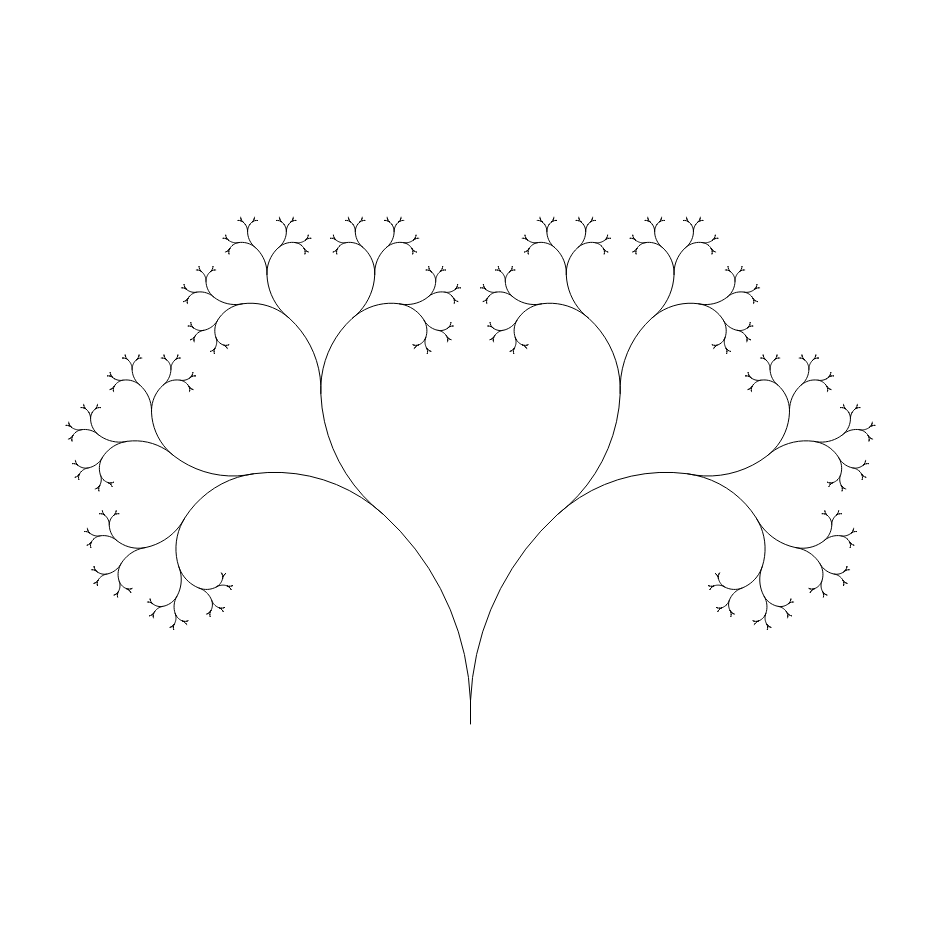### Randomness

In the introduction, we saw that grammars can have multiple non-terminals with the same name. We can use this to introduce an element of randomness into the generated images.

grammar.add_non_terminal('Arc Left', arc_left_segment * 7)


By randomly using shorter or longer arcs, a different tree is generated each time the script is run.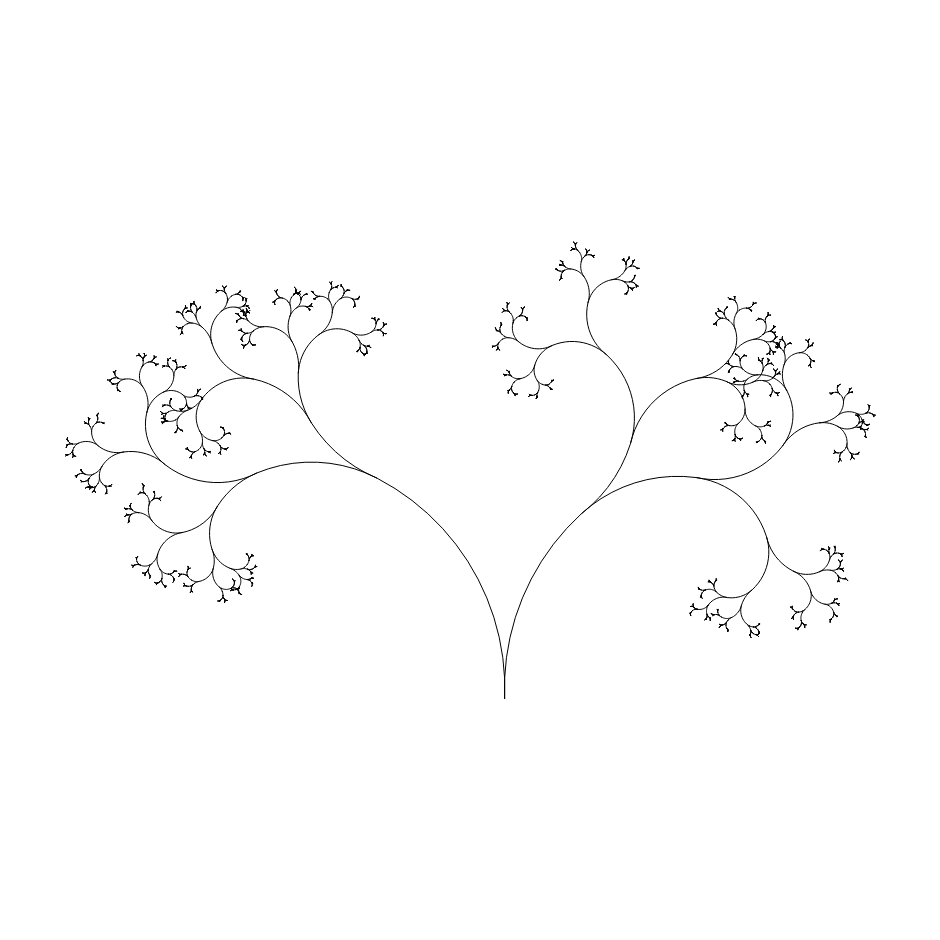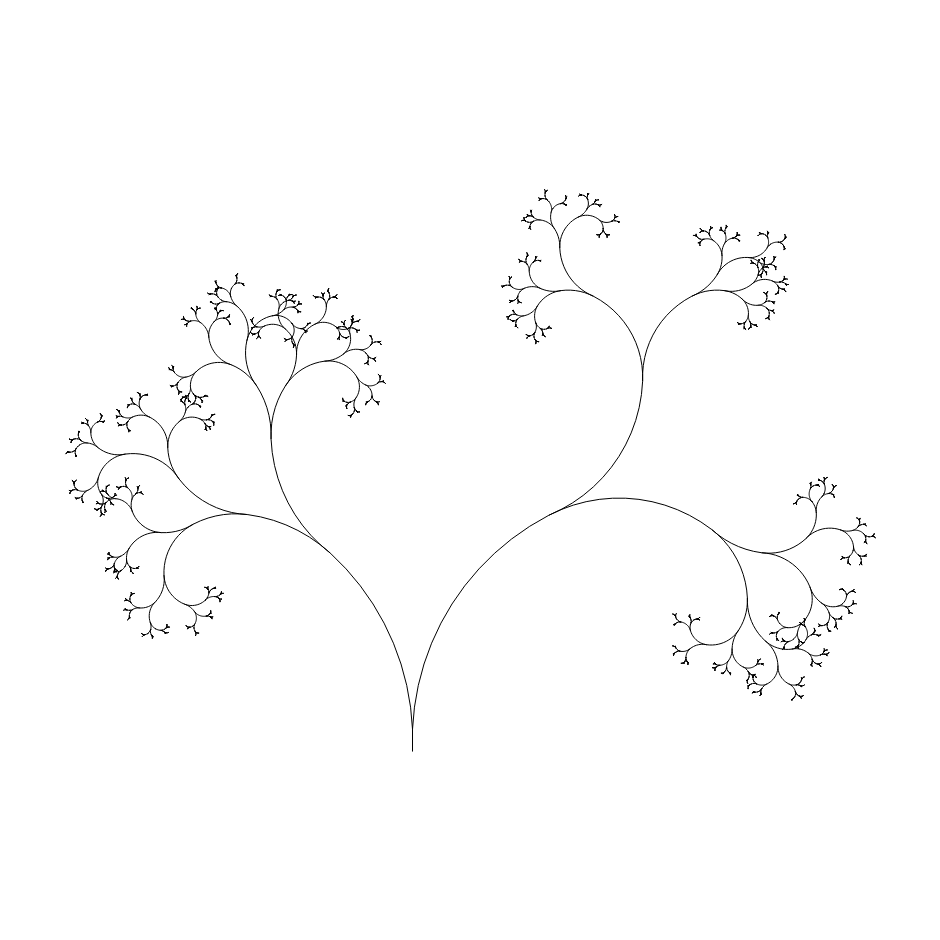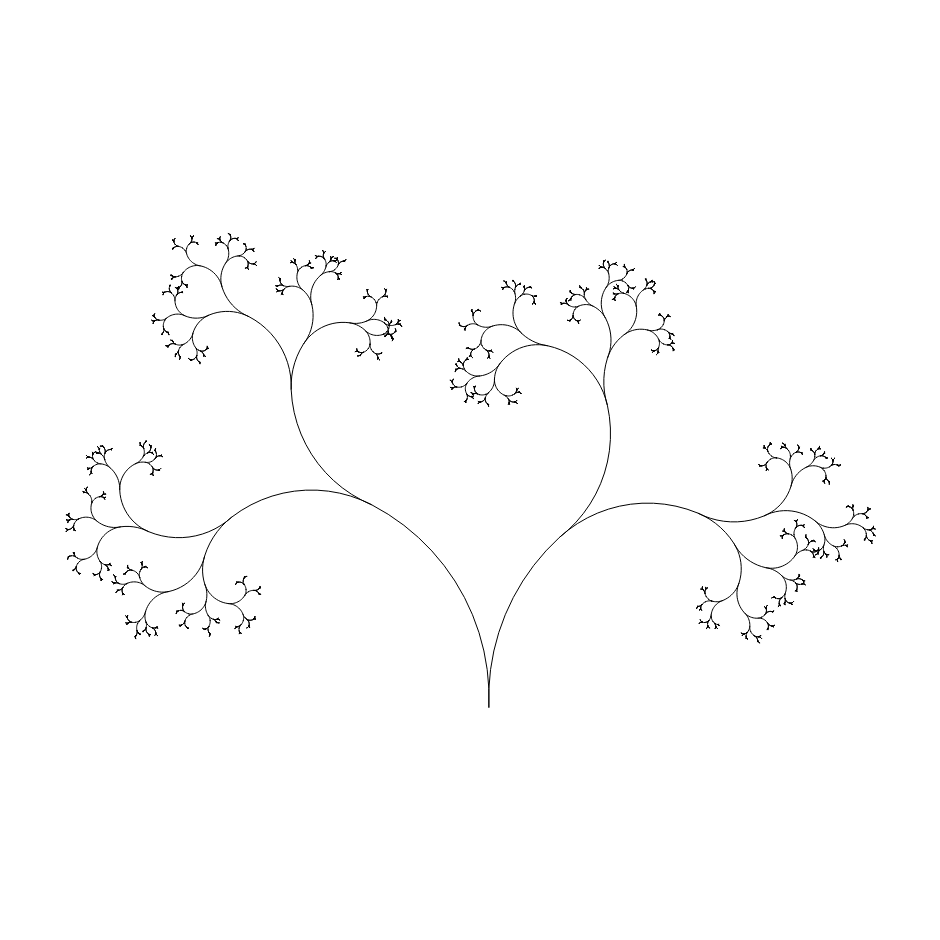## Evolving Rule Sets

Time to explore a different direction from the same starting point, to see how complex grammars can be built with small steps.

### Starting Point

Make the branches straight at an 60deg angle, and grow three "trees" starting from a root in the center.

grammar.add_non_terminal('Branch Right', [
lambda turtle: turtle.forward(1.0),
lambda turtle: turtle.turn_right(60)
])
lambda turtle: turtle.forward(1.0),
lambda turtle: turtle.turn_left(60)
])

'Tree',
lambda turtle: turtle.turn_left(120),
'Tree',
lambda turtle: turtle.turn_left(120),
'Tree',
])

scale = 0.5
lambda turtle: turtle.store(),
'Branch Left',
lambda turtle: turtle.scale(scale),
'Tree',
lambda turtle: turtle.restore(),
# We can't TCO anymore
lambda turtle: turtle.store(),
'Branch Right',
lambda turtle: turtle.scale(scale),
'Tree',
lambda turtle: turtle.restore(),
])


### Randomly Terminating Branches

… by adding an empty "Tree" non-terminal

grammar.add_non_terminal('Tree', [
lambda turtle: turtle.store(),
'Branch Left',
lambda turtle: turtle.scale(scale),
'Tree',
lambda turtle: turtle.restore(),
# We can't TCO anymore
lambda turtle: turtle.store(),
'Branch Right',
lambda turtle: turtle.scale(scale),
'Tree',
lambda turtle: turtle.restore(),
], 8)


### Randomized Scaling

Now extract a "Scale" non-terminal that scales the turtle only 50% of the time.

scale = 0.5
lambda turtle: turtle.scale(scale),
])


### Thick Lines

Using a ThickTurtle, we can draw lines with different widths

from generative_cfg import *

# ...

backend = SVGBackend()
turtle = ThickTurtle(backend)
turtle.thickness = 0.05

grammar.expand('Root', turtle, 12)


and modify scale to adjust the turtles scale, too.

scale = 0.5
lambda turtle: turtle.scale(scale),
lambda turtle: turtle.scale_thickness(0.6),
])


### Decoration

To make the result more visually interesting, we can add random "decoration" elements to the grammar.

grammar.add_non_terminal('Circle', [
lambda turtle: turtle.draw_filled_circle(0.2),
])
lambda turtle: turtle.draw_circle(0.2),
], 2)

grammar.add_non_terminal('Tree', [
lambda turtle: turtle.store(),
'Branch Left',
'Scale',
'Circle',
'Tree',
lambda turtle: turtle.restore(),
lambda turtle: turtle.store(),
'Branch Right',
'Scale',
'Circle',
'Tree',
lambda turtle: turtle.restore(),
], 8)


## Further Work

There are a bunch of things that can be done to extend the ideas presented here.

A simple change would be to add a “finally” function to rules that is executed once the expansion depth has reached some limit. As far as I can tell, this would be equivalent to context-free L-Systems and can be used to draw all kinds of fractals and plants.

The turtle graphics implementation used here is very limited and it would be easy to extend it to support drawing circles, polygons or thick lines.

Another direction could be to implement different kinds of states (besides turtle graphics) that the grammar can operate on. (Hint: Quadtrees and Octrees are one possibility).

If you have any questions about how this works, how to implement it the programming language of your choice, or if you want to share an image you generated, feel free to contact me at cfg <at> leonrische.me

## References

Turtle Graphics are a great way to teach programming to children, if you are interested in that angle, I recommend the book “Mindstorms: Children, Computers and Powerful Ideas” by Seymour Papert.

For some background on the LOGO language and its history, visit https://el.media.mit.edu/logo-foundation/what_is_logo/index.html

With a few small changes, the context free grammars described in this article can be expanded to context-free L-Systems which can be used to model the growth of different kinds of plants. The book “The Algorithmic Beauty of Plants” is a great introduction into this topic and can be downloaded for free at http://algorithmicbotany.org/papers/.

Context Free Art is a program generates (colored) images from CFGs, the website contains a large collection of example programs / images.